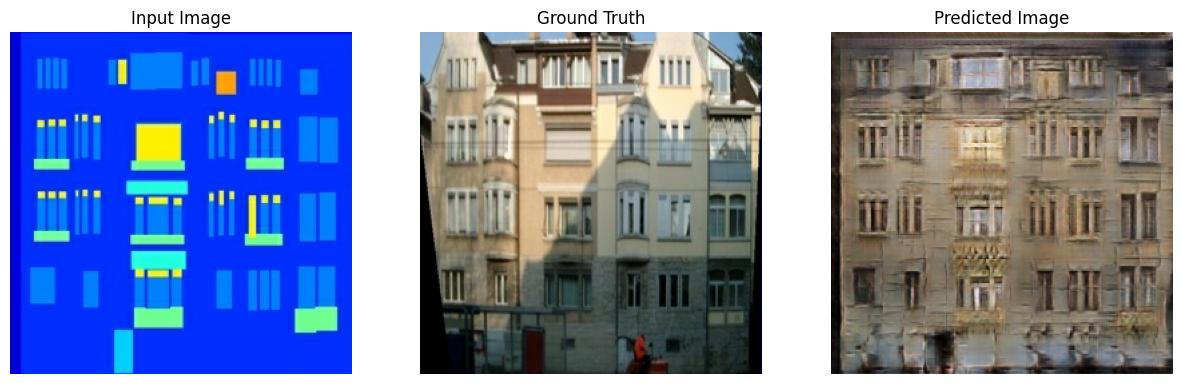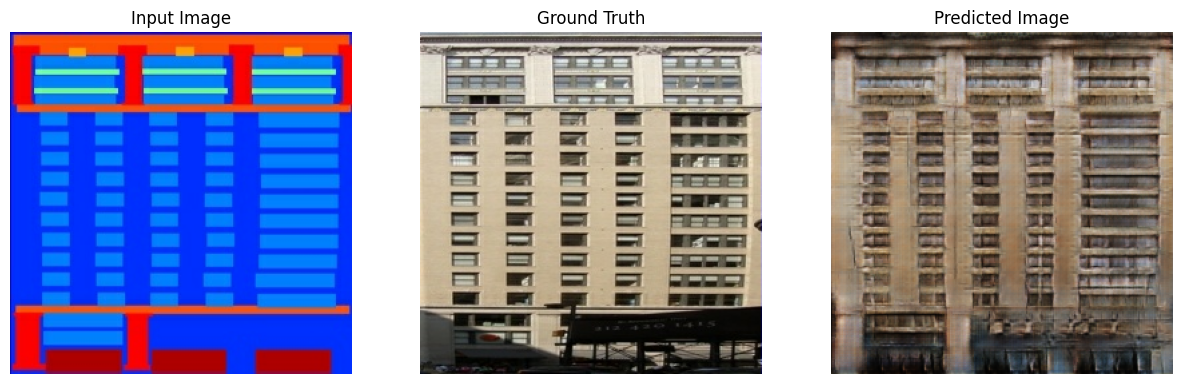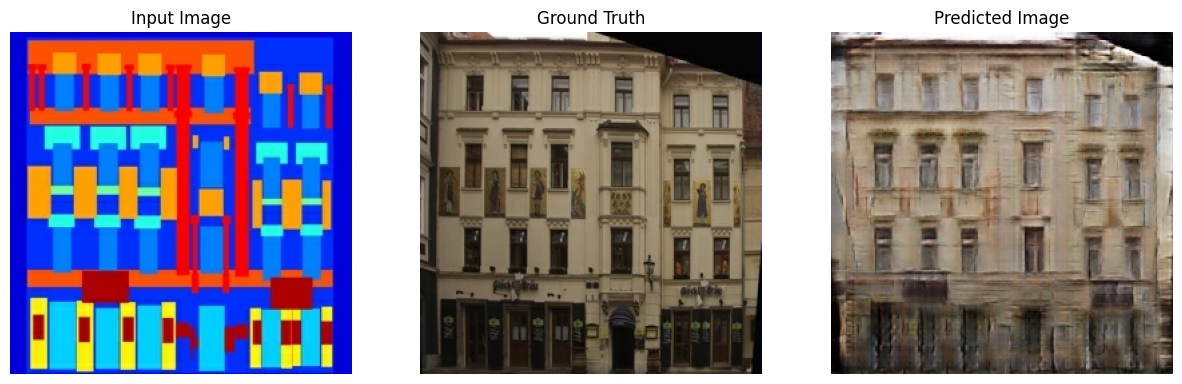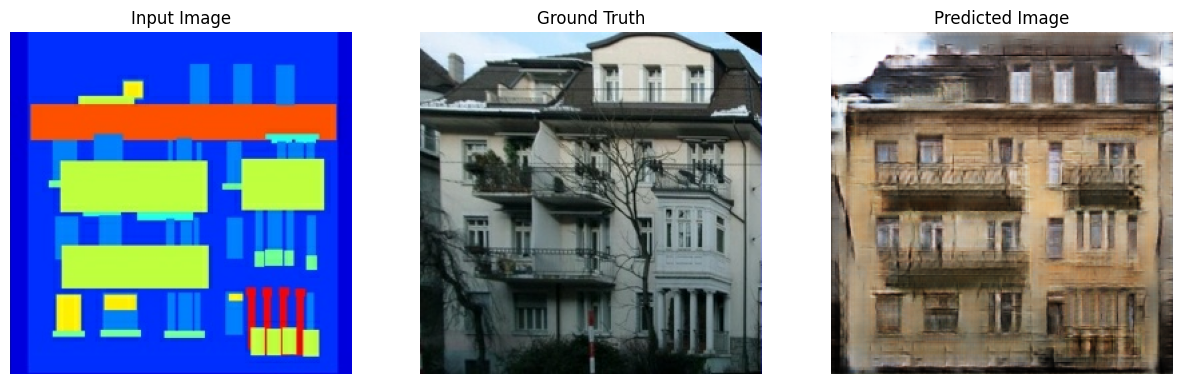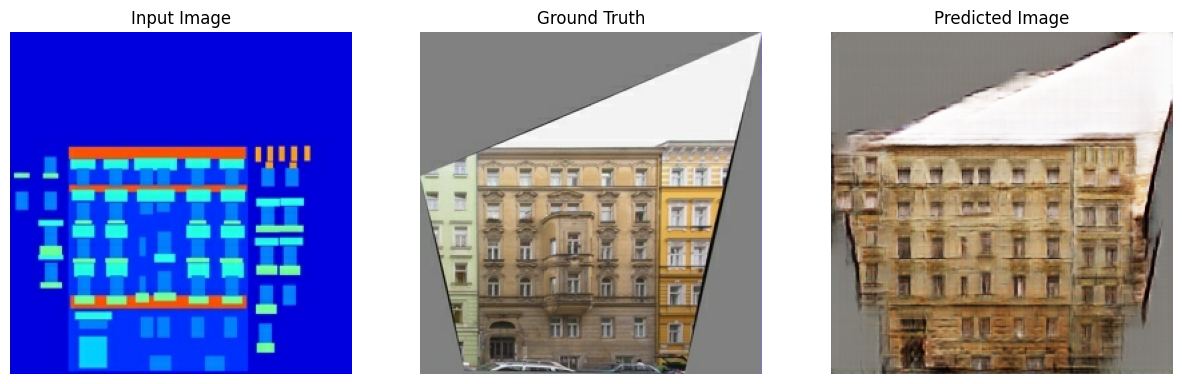Join us at TensorFlow World, Oct 28-31. Use code TF20 for 20% off select passes.

# Pix2Pix

This notebook demonstrates image to image translation using conditional GAN's, as described in Image-to-Image Translation with Conditional Adversarial Networks. Using this technique we can colorize black and white photos, convert google maps to google earth, etc. Here, we convert building facades to real buildings.

In example, we will use the CMP Facade Database, helpfully provided by the Center for Machine Perception at the Czech Technical University in Prague. To keep our example short, we will use a preprocessed copy of this dataset, created by the authors of the paper above.

Each epoch takes around 15 seconds on a single V100 GPU.

Below is the output generated after training the model for 200 epochs.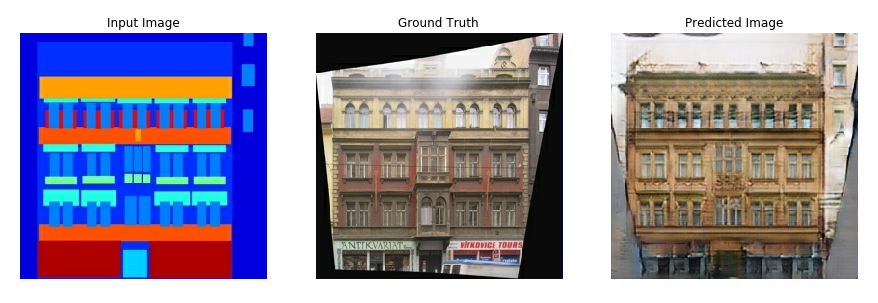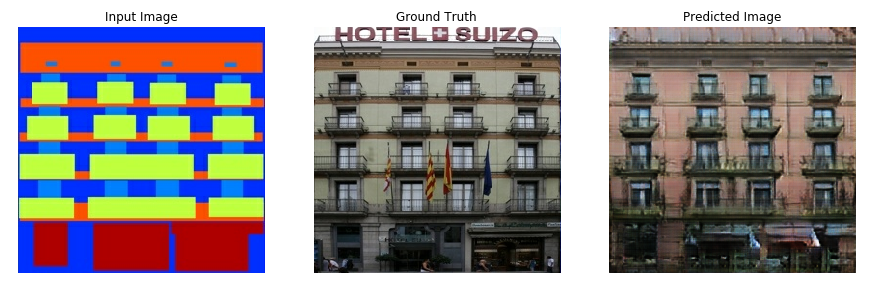## Import TensorFlow and other libraries

``````from __future__ import absolute_import, division, print_function, unicode_literals

try:
# %tensorflow_version only exists in Colab.
%tensorflow_version 2.x
except Exception:
pass
import tensorflow as tf

import os
import time
import matplotlib.pyplot as plt
from IPython.display import clear_output
``````
```ERROR: tensorflow-gpu 2.0.0b1 has requirement tb-nightly<1.14.0a20190604,>=1.14.0a20190603, but you'll have tb-nightly 1.15.0a20190816 which is incompatible.
```

## Load the dataset

You can download this dataset and similar datasets from here. As mentioned in the paper we apply random jittering and mirroring to the training dataset.

• In random jittering, the image is resized to `286 x 286` and then randomly cropped to `256 x 256`
• In random mirroring, the image is randomly flipped horizontally i.e left to right.
``````_URL = 'https://people.eecs.berkeley.edu/~tinghuiz/projects/pix2pix/datasets/facades.tar.gz'

origin=_URL,
extract=True)

PATH = os.path.join(os.path.dirname(path_to_zip), 'facades/')
``````
```Downloading data from https://people.eecs.berkeley.edu/~tinghuiz/projects/pix2pix/datasets/facades.tar.gz
30171136/30168306 [==============================] - 2s 0us/step
```
``````BUFFER_SIZE = 400
BATCH_SIZE = 1
IMG_WIDTH = 256
IMG_HEIGHT = 256
``````
``````def load(image_file):
image = tf.image.decode_jpeg(image)

w = tf.shape(image)

w = w // 2
real_image = image[:, :w, :]
input_image = image[:, w:, :]

input_image = tf.cast(input_image, tf.float32)
real_image = tf.cast(real_image, tf.float32)

return input_image, real_image
``````
``````inp, re = load(PATH+'train/100.jpg')
# casting to int for matplotlib to show the image
plt.figure()
plt.imshow(inp/255.0)
plt.figure()
plt.imshow(re/255.0)
``````
`<matplotlib.image.AxesImage at 0x7fe1bc8d2550>`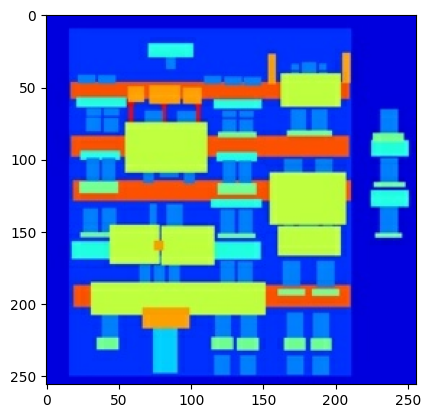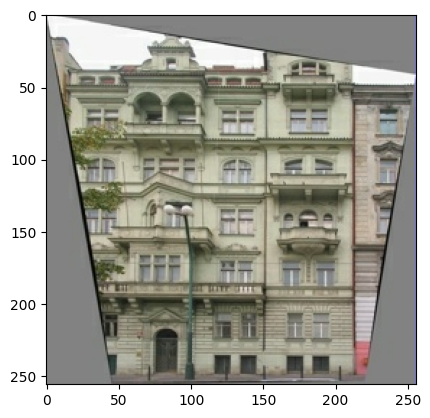``````def resize(input_image, real_image, height, width):
input_image = tf.image.resize(input_image, [height, width],
method=tf.image.ResizeMethod.NEAREST_NEIGHBOR)
real_image = tf.image.resize(real_image, [height, width],
method=tf.image.ResizeMethod.NEAREST_NEIGHBOR)

return input_image, real_image
``````
``````def random_crop(input_image, real_image):
stacked_image = tf.stack([input_image, real_image], axis=0)
cropped_image = tf.image.random_crop(
stacked_image, size=[2, IMG_HEIGHT, IMG_WIDTH, 3])

return cropped_image, cropped_image
``````
``````# normalizing the images to [-1, 1]

def normalize(input_image, real_image):
input_image = (input_image / 127.5) - 1
real_image = (real_image / 127.5) - 1

return input_image, real_image
``````
``````@tf.function()
def random_jitter(input_image, real_image):
# resizing to 286 x 286 x 3
input_image, real_image = resize(input_image, real_image, 286, 286)

# randomly cropping to 256 x 256 x 3
input_image, real_image = random_crop(input_image, real_image)

if tf.random.uniform(()) > 0.5:
# random mirroring
input_image = tf.image.flip_left_right(input_image)
real_image = tf.image.flip_left_right(real_image)

return input_image, real_image
``````
``````# As you can see in the images below
# that they are going through random jittering
# Random jittering as described in the paper is to
# 1. Resize an image to bigger height and width
# 2. Randomnly crop to the original size
# 3. Randomnly flip the image horizontally

plt.figure(figsize=(6, 6))
for i in range(4):
rj_inp, rj_re = random_jitter(inp, re)
plt.subplot(2, 2, i+1)
plt.imshow(rj_inp/255.0)
plt.axis('off')
plt.show()
``````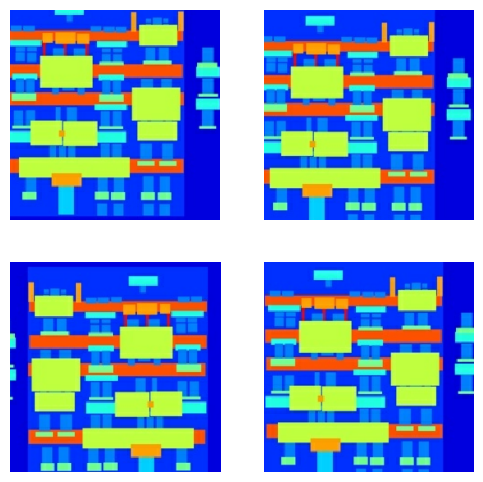``````def load_image_train(image_file):
input_image, real_image = load(image_file)
input_image, real_image = random_jitter(input_image, real_image)
input_image, real_image = normalize(input_image, real_image)

return input_image, real_image
``````
``````def load_image_test(image_file):
input_image, real_image = load(image_file)
input_image, real_image = resize(input_image, real_image,
IMG_HEIGHT, IMG_WIDTH)
input_image, real_image = normalize(input_image, real_image)

return input_image, real_image
``````

## Input Pipeline

``````train_dataset = tf.data.Dataset.list_files(PATH+'train/*.jpg')
num_parallel_calls=tf.data.experimental.AUTOTUNE)
train_dataset = train_dataset.cache().shuffle(BUFFER_SIZE)
train_dataset = train_dataset.batch(1)
``````
``````test_dataset = tf.data.Dataset.list_files(PATH+'test/*.jpg')
test_dataset = test_dataset.batch(1)
``````

## Build the Generator

• The architecture of generator is a modified U-Net.
• Each block in the encoder is (Conv -> Batchnorm -> Leaky ReLU)
• Each block in the decoder is (Transposed Conv -> Batchnorm -> Dropout(applied to the first 3 blocks) -> ReLU)
• There are skip connections between the encoder and decoder (as in U-Net).
``````OUTPUT_CHANNELS = 3
``````
``````def downsample(filters, size, apply_batchnorm=True):
initializer = tf.random_normal_initializer(0., 0.02)

result = tf.keras.Sequential()
tf.keras.layers.Conv2D(filters, size, strides=2, padding='same',
kernel_initializer=initializer, use_bias=False))

if apply_batchnorm:

return result
``````
``````down_model = downsample(3, 4)
down_result = down_model(tf.expand_dims(inp, 0))
print (down_result.shape)
``````
```(1, 128, 128, 3)
```
``````def upsample(filters, size, apply_dropout=False):
initializer = tf.random_normal_initializer(0., 0.02)

result = tf.keras.Sequential()
tf.keras.layers.Conv2DTranspose(filters, size, strides=2,
kernel_initializer=initializer,
use_bias=False))

if apply_dropout:

return result
``````
``````up_model = upsample(3, 4)
up_result = up_model(down_result)
print (up_result.shape)
``````
```(1, 256, 256, 3)
```
``````def Generator():
down_stack = [
downsample(64, 4, apply_batchnorm=False), # (bs, 128, 128, 64)
downsample(128, 4), # (bs, 64, 64, 128)
downsample(256, 4), # (bs, 32, 32, 256)
downsample(512, 4), # (bs, 16, 16, 512)
downsample(512, 4), # (bs, 8, 8, 512)
downsample(512, 4), # (bs, 4, 4, 512)
downsample(512, 4), # (bs, 2, 2, 512)
downsample(512, 4), # (bs, 1, 1, 512)
]

up_stack = [
upsample(512, 4, apply_dropout=True), # (bs, 2, 2, 1024)
upsample(512, 4, apply_dropout=True), # (bs, 4, 4, 1024)
upsample(512, 4, apply_dropout=True), # (bs, 8, 8, 1024)
upsample(512, 4), # (bs, 16, 16, 1024)
upsample(256, 4), # (bs, 32, 32, 512)
upsample(128, 4), # (bs, 64, 64, 256)
upsample(64, 4), # (bs, 128, 128, 128)
]

initializer = tf.random_normal_initializer(0., 0.02)
last = tf.keras.layers.Conv2DTranspose(OUTPUT_CHANNELS, 4,
strides=2,
kernel_initializer=initializer,
activation='tanh') # (bs, 256, 256, 3)

concat = tf.keras.layers.Concatenate()

inputs = tf.keras.layers.Input(shape=[None,None,3])
x = inputs

# Downsampling through the model
skips = []
for down in down_stack:
x = down(x)
skips.append(x)

skips = reversed(skips[:-1])

# Upsampling and establishing the skip connections
for up, skip in zip(up_stack, skips):
x = up(x)
x = concat([x, skip])

x = last(x)

return tf.keras.Model(inputs=inputs, outputs=x)
``````
``````generator = Generator()

gen_output = generator(inp[tf.newaxis,...], training=False)
plt.imshow(gen_output[0,...])
``````
```WARNING: Logging before flag parsing goes to stderr.
W0817 00:55:36.569016 140608053683968 image.py:648] Clipping input data to the valid range for imshow with RGB data ([0..1] for floats or [0..255] for integers).

<matplotlib.image.AxesImage at 0x7fe104104c18>```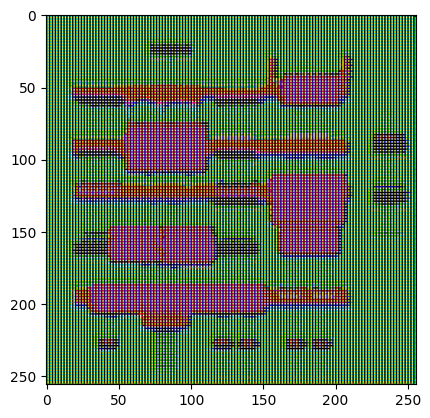## Build the Discriminator

• The Discriminator is a PatchGAN.
• Each block in the discriminator is (Conv -> BatchNorm -> Leaky ReLU)
• The shape of the output after the last layer is (batch_size, 30, 30, 1)
• Each 30x30 patch of the output classifies a 70x70 portion of the input image (such an architecture is called a PatchGAN).
• Discriminator receives 2 inputs.
• Input image and the target image, which it should classify as real.
• Input image and the generated image (output of generator), which it should classify as fake.
• We concatenate these 2 inputs together in the code (`tf.concat([inp, tar], axis=-1)`)
``````def Discriminator():
initializer = tf.random_normal_initializer(0., 0.02)

inp = tf.keras.layers.Input(shape=[None, None, 3], name='input_image')
tar = tf.keras.layers.Input(shape=[None, None, 3], name='target_image')

x = tf.keras.layers.concatenate([inp, tar]) # (bs, 256, 256, channels*2)

down1 = downsample(64, 4, False)(x) # (bs, 128, 128, 64)
down2 = downsample(128, 4)(down1) # (bs, 64, 64, 128)
down3 = downsample(256, 4)(down2) # (bs, 32, 32, 256)

zero_pad1 = tf.keras.layers.ZeroPadding2D()(down3) # (bs, 34, 34, 256)
conv = tf.keras.layers.Conv2D(512, 4, strides=1,
kernel_initializer=initializer,
use_bias=False)(zero_pad1) # (bs, 31, 31, 512)

batchnorm1 = tf.keras.layers.BatchNormalization()(conv)

leaky_relu = tf.keras.layers.LeakyReLU()(batchnorm1)

zero_pad2 = tf.keras.layers.ZeroPadding2D()(leaky_relu) # (bs, 33, 33, 512)

last = tf.keras.layers.Conv2D(1, 4, strides=1,
kernel_initializer=initializer)(zero_pad2) # (bs, 30, 30, 1)

return tf.keras.Model(inputs=[inp, tar], outputs=last)
``````
``````discriminator = Discriminator()
disc_out = discriminator([inp[tf.newaxis,...], gen_output], training=False)
plt.imshow(disc_out[0,...,-1], vmin=-20, vmax=20, cmap='RdBu_r')
plt.colorbar()
``````
`<matplotlib.colorbar.Colorbar at 0x7fe0ff6e95c0>`To learn more about the architecture and the hyperparameters you can refer the paper.

## Define the loss functions and the optimizer

• Discriminator loss

• The discriminator loss function takes 2 inputs; real images, generated images
• real_loss is a sigmoid cross entropy loss of the real images and an array of ones(since these are the real images)
• generated_loss is a sigmoid cross entropy loss of the generated images and an array of zeros(since these are the fake images)
• Then the total_loss is the sum of real_loss and the generated_loss
• Generator loss

• It is a sigmoid cross entropy loss of the generated images and an array of ones.
• The paper also includes L1 loss which is MAE (mean absolute error) between the generated image and the target image.
• This allows the generated image to become structurally similar to the target image.
• The formula to calculate the total generator loss = gan_loss + LAMBDA * l1_loss, where LAMBDA = 100. This value was decided by the authors of the paper.
``````LAMBDA = 100
``````
``````loss_object = tf.keras.losses.BinaryCrossentropy(from_logits=True)
``````
``````def discriminator_loss(disc_real_output, disc_generated_output):
real_loss = loss_object(tf.ones_like(disc_real_output), disc_real_output)

generated_loss = loss_object(tf.zeros_like(disc_generated_output), disc_generated_output)

total_disc_loss = real_loss + generated_loss

``````
``````def generator_loss(disc_generated_output, gen_output, target):
gan_loss = loss_object(tf.ones_like(disc_generated_output), disc_generated_output)

# mean absolute error
l1_loss = tf.reduce_mean(tf.abs(target - gen_output))

total_gen_loss = gan_loss + (LAMBDA * l1_loss)

``````
``````generator_optimizer = tf.keras.optimizers.Adam(2e-4, beta_1=0.5)
discriminator_optimizer = tf.keras.optimizers.Adam(2e-4, beta_1=0.5)
``````

## Checkpoints (Object-based saving)

``````checkpoint_dir = './training_checkpoints'
checkpoint_prefix = os.path.join(checkpoint_dir, "ckpt")
checkpoint = tf.train.Checkpoint(generator_optimizer=generator_optimizer,
discriminator_optimizer=discriminator_optimizer,
generator=generator,
discriminator=discriminator)
``````

## Training

• We start by iterating over the dataset
• The generator gets the input image and we get a generated output.
• The discriminator receives the input_image and the generated image as the first input. The second input is the input_image and the target_image.
• Next, we calculate the generator and the discriminator loss.
• Then, we calculate the gradients of loss with respect to both the generator and the discriminator variables(inputs) and apply those to the optimizer.
• This entire procedure is shown in the images below.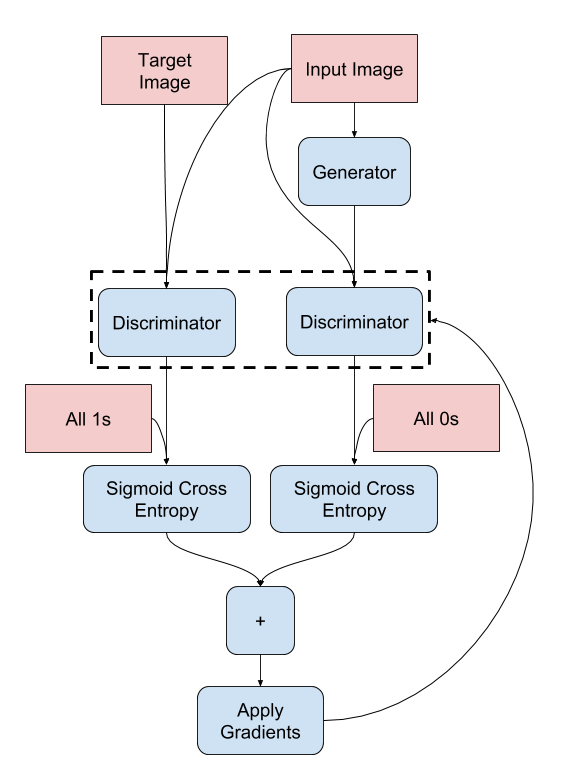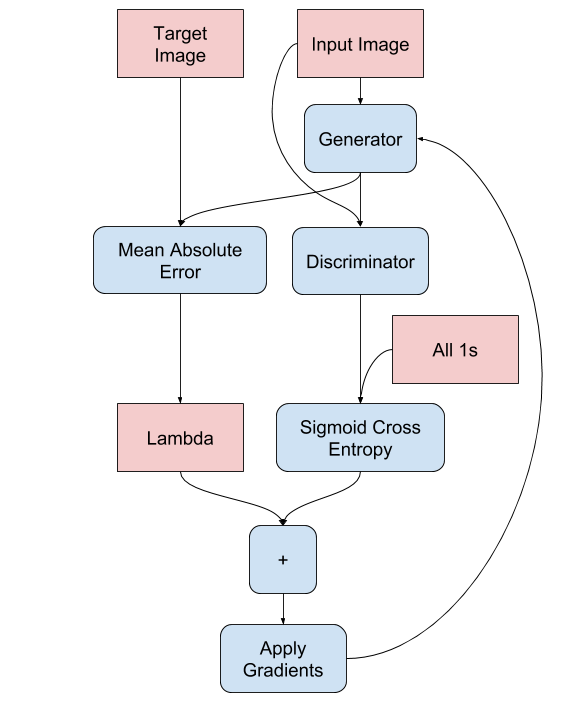## Generate Images

• After training, its time to generate some images!
• We pass images from the test dataset to the generator.
• The generator will then translate the input image into the output we expect.
• Last step is to plot the predictions and voila!
``````EPOCHS = 150
``````
``````def generate_images(model, test_input, tar):
# the training=True is intentional here since
# we want the batch statistics while running the model
# on the test dataset. If we use training=False, we will get
# the accumulated statistics learned from the training dataset
# (which we don't want)
prediction = model(test_input, training=True)
plt.figure(figsize=(15,15))

display_list = [test_input, tar, prediction]
title = ['Input Image', 'Ground Truth', 'Predicted Image']

for i in range(3):
plt.subplot(1, 3, i+1)
plt.title(title[i])
# getting the pixel values between [0, 1] to plot it.
plt.imshow(display_list[i] * 0.5 + 0.5)
plt.axis('off')
plt.show()
``````
``````@tf.function
def train_step(input_image, target):
with tf.GradientTape() as gen_tape, tf.GradientTape() as disc_tape:
gen_output = generator(input_image, training=True)

disc_real_output = discriminator([input_image, target], training=True)
disc_generated_output = discriminator([input_image, gen_output], training=True)

gen_loss = generator_loss(disc_generated_output, gen_output, target)
disc_loss = discriminator_loss(disc_real_output, disc_generated_output)

generator.trainable_variables)
discriminator.trainable_variables)

generator.trainable_variables))
discriminator.trainable_variables))
``````
``````def fit(train_ds, epochs, test_ds):
for epoch in range(epochs):
start = time.time()

# Train
for input_image, target in train_ds:
train_step(input_image, target)

clear_output(wait=True)
# Test on the same image so that the progress of the model can be
# easily seen.
for example_input, example_target in test_ds.take(1):
generate_images(generator, example_input, example_target)

# saving (checkpoint) the model every 20 epochs
if (epoch + 1) % 20 == 0:
checkpoint.save(file_prefix = checkpoint_prefix)

print ('Time taken for epoch {} is {} sec\n'.format(epoch + 1,
time.time()-start))
``````
``````fit(train_dataset, EPOCHS, test_dataset)
`````````Time taken for epoch 150 is 14.198184490203857 sec

```

## Restore the latest checkpoint and test

``````!ls {checkpoint_dir}
``````
```checkpoint          ckpt-4.data-00001-of-00002
ckpt-1.data-00000-of-00002  ckpt-4.index
ckpt-1.data-00001-of-00002  ckpt-5.data-00000-of-00002
ckpt-1.index            ckpt-5.data-00001-of-00002
ckpt-2.data-00000-of-00002  ckpt-5.index
ckpt-2.data-00001-of-00002  ckpt-6.data-00000-of-00002
ckpt-2.index            ckpt-6.data-00001-of-00002
ckpt-3.data-00000-of-00002  ckpt-6.index
ckpt-3.data-00001-of-00002  ckpt-7.data-00000-of-00002
ckpt-3.index            ckpt-7.data-00001-of-00002
ckpt-4.data-00000-of-00002  ckpt-7.index
```
``````# restoring the latest checkpoint in checkpoint_dir
checkpoint.restore(tf.train.latest_checkpoint(checkpoint_dir))
``````
`<tensorflow.python.training.tracking.util.CheckpointLoadStatus at 0x7fdc946df630>`

## Generate using test dataset

``````# Run the trained model on the entire test dataset
for inp, tar in test_dataset.take(5):
generate_images(generator, inp, tar)
``````